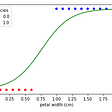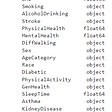# Requirements

• Some basic Python knowledge, syntax and such.

# Data

`!wget https://download.microsoft.com/download/3/E/1/3E1C3F21-ECDB-4869-8368-6DEBA77B919F/kagglecatsanddogs_3367a.zip!unzip kagglecatsanddogs_3367a.zip -d data`
`data||__PetImages|  ||  |__Cat (directory of cat images|  ||  |__Dog (directory of dog images)||__MSR-LA - 3467.docx||__readme.txt`

## Cleaning the Data

`import osfrom os import pathimport PILrm_count, count = 0, 0for dir in ("Cat", "Dog"):    dest = path.join("data/PetImages", dir)    files = os.listdir(dest)    for fname in files:        full = path.join(dest, fname)        if fname.find("jpg") == -1:            os.remove(full)            rm_count += 1            continue        try:            PIL.Image.open(full).convert("RGB")        except PIL.UnidentifiedImageError:            os.remove(full)            rm_count += 1            continue        count += 1print("Kept %d files, removed %d files" % (count, rm_count))`

`from torch.utils.data import Datasetfrom torchvision import transformsimport PILimport osclass CatDogDataset(Dataset):    def __init__(self, ...):        pass        def __len__(self):        pass        def __getitem__(self, idx):        pass`
`def __init__(self, datapath, custom_transforms = []):    # count images in examples    self.datapath = datapath    self.cat_fnames = os.listdir( os.path.join(datapath, "Cat") )    self.dog_fnames = os.listdir( os.path.join(datapath, "Dog") )    self.transforms = transforms.Compose([        transforms.Resize(96),        transforms.CenterCrop(96),        *custom_transforms,        transforms.ToTensor()    ])`
`def __len__(self):    return len(self.cat_fnames) + len(self.dog_fnames)`
`def __getitem__(self, idx):    # lower indices get cats while higher indices get dogs    impath = None    is_cat = idx < len(self.cat_fnames)    if is_cat:        fname = self.cat_fnames[idx]        impath = os.path.join(self.datapath, "Cat", fname)    else:        fname = self.dog_fnames[idx - len(self.cat_fnames)]        impath = os.path.join(self.datapath, "Dog", fname)    # open image and apply transforms    image = PIL.Image.open(impath).convert("RGB")    as_tensor = self.transforms(image)    # return image as tensor and binary label for cat (0) / dog (1)    return as_tensor, int(not is_cat)`
`from torch.utils.data import DataLoaderdataset = CatDogDataset("data/PetImages")data = DataLoader(dataset, batch_size=128, shuffle=True)`
`from torch.utils.data import DataLoader, random_splitdef load_data(datapath):    dataset = CatDogDataset(datapath)    # We pass in our dataset and the split distribution to get our    # subsets in a tuple.    train_set, test_set = random_split(dataset, (19998, 5000))    train_data, test_data = (        DataLoader(train_set, batch_size=128, shuffle=True),         DataLoader(test_set, batch_size=128, shuffle=True)    )`

# The Model

`import torchimport torchvisionmodel = torchvision.models.resnet18(pretrained=False)`
`print(net.fc)> Linear(in_features=512, out_features=1000, bias=True)`
`model.fc = torch.nn.Linear(512, 2)`

# Training

`train_data, test_data = load_data("data/PetImages")`
`device = torch.device("cuda" if torch.cuda.is_available() else "cpu")model = model.to(device)`
`loss_func = torch.nn.CrossEntropyLoss().to(device)optim = torch.optim.Adam(model.parameters(), lr=1e-3).to(device)`
`training_accuracies = []testing_accuracies = []losses = []`
`for epoch in range(20):    # set model in training mode    model.train()    for images, labels in train_data:        # get data on GPU and make prediction        images, labels = images.to(device), labels.to(device)        preds = model(images)        # calculate loss and training accuracy from prediction        loss = loss_func(preds, labels)        acc = (preds.argmax(1) == labels).float().mean()        # calculate gradient and update model parameters        optim.zero_grad() # reset gradients in optimizer        loss.backward()   # calculate new gradients for parameters         optim.step()      # add gradients to parameters        # record metrics        train_accuracies.append(acc.item())        losses.append(loss.item())    # set model in test mode    model.eval()    for images, labels in test_data:        # get data on GPU and make prediction        images, labels = images.to(device), labels.to(device)        preds = model(images)        # calculate and record accuracy         acc = (preds.argmax(1) == labels).float().mean()        test_accuracies.append(acc.item())`
`import matplotlib.pyplot as pltfig, (ax1, ax2, ax3) = plt.subplots(1, 3)fig.suptitle("Visualization")ax1.set_title("Training Loss")ax1.set_ylim([0, 1])ax1.plot(range(len(losses)), losses)ax2.set_title("Training Accuracy")ax2.set_ylim([0, 1])ax2.plot(range(len(train_accuracies)), train_accuracies)ax3.set_title("Test Accuracy")ax3.set_ylim([0, 1])ax3.plot(range(len(test_accuracies)), test_accuracies)plt.show()`

# Conclusion

• Data augmentations in the training data: RandomHorizontalFlip, RandomCrop, ColorJitter to name a few
• Tuning hyper-parameters: learning rate, epochs trained, batch size, weight_decay, etc.
• Use a different model: a ResNet variant with more layers, GoogleNet, DenseNet. Or you can try to implement your own Convolutional Neural Network for this task.

--

--

--

## More from Larry Win

UT Austin CS Major - Spring 2022

Love podcasts or audiobooks? Learn on the go with our new app.

## Zero-Shot Text Classification & Evaluation## The definitive guide to Accuracy, Precision, and Recall for product developers## Sales Prediction — Rossmann Pharmaceuticals## Word Embeddings Explained## CoC Episode 4: An Introduction to Feature Engineering for Machine Learning## Larry Win

UT Austin CS Major - Spring 2022

## Converting multiple columns ,object (binary — yes , no) type to integer in Python: